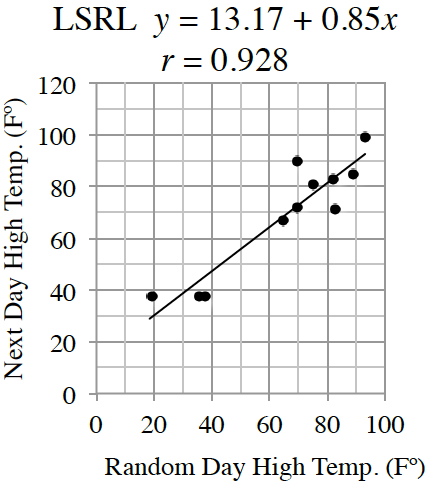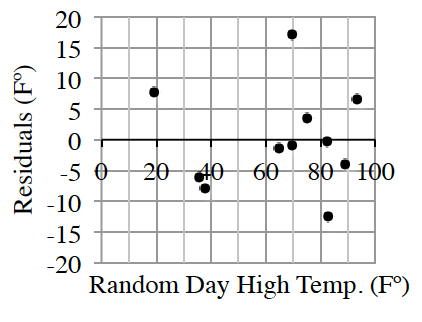### Home > CCA > Chapter 8 > Lesson 8.1.5 > Problem8-54

8-54.

Mitchell likes to study the weather. He is fascinated by the sophistication of the computer models used to make weather predictions. Mitchell wonders if he can make his own model to predict the next day’s high temperature in his area based only on today’s high temperature. He selects $11$ days at random and gets the temperatures from the Internet. The results from his computer spreadsheet follow.Random Day $(\text{F}^{\large \circ })$

Next Day $(\text{F}^{\large \circ })$

$93.2$

$99$

$69.8$

$71.6$

$82.9$

$71.1$

$82.4$

$82.9$

$19.4$

$37.4$

$69.8$

$89.6$

$35.6$

$37.4$

$89.1$

$84.9$

$37.9$

$37.4$

$75.2$

$80.6$

$64.9$

$66.9$

1. Write a few sentences that describe the association. Remember to include interpretations of slope and $R^2$.

2. Use the graph to estimate the largest residual. To what point does it belong?

The largest residual belongs to the day after the $69.8$ degree day with a value of about $17$ degrees.

3. Using the LSRL model, estimate tomorrow’s high temperature based on today’s high temperature of $55$ degrees in Mitchell’s area. Use appropriate precision.

Let $x = 55$ in the given LSRL equation.

$60$ degrees

4. Consider the upper and lower bounds of the prediction Mitchell made in part (c) above. Is Mitchell’s model ready to replace the complex models of the professional meteorologists? Support your answer.

Draw upper and lover boundary lines on the LSRL graph. How far apart are these lines?